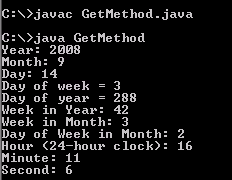Tutorials

# Java Get Method

In this example you will learn how to use the get method in Java and video tutorial you will learn How to get day month and year from date in Java?

In this example you will learn how to use the get method in Java and video tutorial you will learn How to get day month and year from date in Java?

# Java Get Method

In this example you will learn how to use the get method in Java.

Java provides various methods and functions that makes this language easy to learn and use. So just go through the below given example code to see when and how to use get method in Java.

The example is going to show the date details.. including year, date and month etc.. with the use of get method.

Here is the video tutorial of "How to get day month and year from date in Java?":

Code for Java Get Method

 ``` import java.util.Calendar; public class GetMethod  {  public static void main(String[] av) {  Calendar cal = Calendar.getInstance();    System.out.println("Year: " + cal.get(Calendar.YEAR));   System.out.println("Month: " + cal.get(Calendar.MONTH));   System.out.println("Day: " + cal.get(Calendar.DAY_OF_MONTH));   System.out.println("Day of week = " + cal.get(Calendar.DAY_OF_WEEK));   System.out.println("Day of year = " + cal.get(Calendar.DAY_OF_YEAR));   System.out.println("Week in Year: " + cal.get(Calendar.WEEK_OF_YEAR));   System.out.println("Week in Month: " + cal.get(Calendar.WEEK_OF_MONTH));   System.out.println("Day of Week in Month: "   + cal.get(Calendar.DAY_OF_WEEK_IN_MONTH));   System.out.println("Hour (24-hour clock): "   + cal.get(Calendar.HOUR_OF_DAY));   System.out.println("Minute: " + cal.get(Calendar.MINUTE));   System.out.println("Second: " + cal.get(Calendar.SECOND));   } }```

Output will be displayed as: